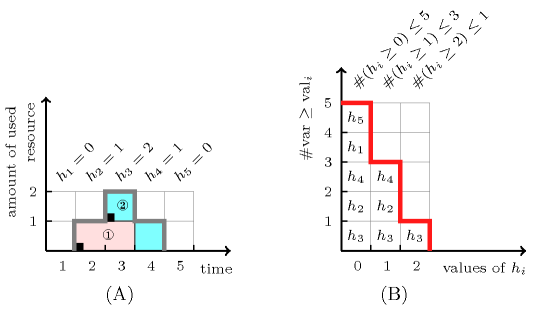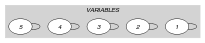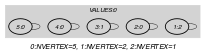## 5.308. ordered_global_cardinality

Origin
Constraint

$\mathrm{𝚘𝚛𝚍𝚎𝚛𝚎𝚍}_\mathrm{𝚐𝚕𝚘𝚋𝚊𝚕}_\mathrm{𝚌𝚊𝚛𝚍𝚒𝚗𝚊𝚕𝚒𝚝𝚢}\left(\mathrm{𝚅𝙰𝚁𝙸𝙰𝙱𝙻𝙴𝚂},\mathrm{𝚅𝙰𝙻𝚄𝙴𝚂}\right)$

Usual name

$\mathrm{𝚘𝚛𝚍𝚐𝚌𝚌}$

Synonym

$\mathrm{𝚘𝚛𝚍𝚎𝚛𝚎𝚍}_\mathrm{𝚐𝚌𝚌}$.

Arguments
 $\mathrm{𝚅𝙰𝚁𝙸𝙰𝙱𝙻𝙴𝚂}$ $\mathrm{𝚌𝚘𝚕𝚕𝚎𝚌𝚝𝚒𝚘𝚗}\left(\mathrm{𝚟𝚊𝚛}-\mathrm{𝚍𝚟𝚊𝚛}\right)$ $\mathrm{𝚅𝙰𝙻𝚄𝙴𝚂}$ $\mathrm{𝚌𝚘𝚕𝚕𝚎𝚌𝚝𝚒𝚘𝚗}\left(\mathrm{𝚟𝚊𝚕}-\mathrm{𝚒𝚗𝚝},\mathrm{𝚘𝚖𝚊𝚡}-\mathrm{𝚒𝚗𝚝}\right)$
Restrictions
 $\mathrm{𝚛𝚎𝚚𝚞𝚒𝚛𝚎𝚍}$$\left(\mathrm{𝚅𝙰𝚁𝙸𝙰𝙱𝙻𝙴𝚂},\mathrm{𝚟𝚊𝚛}\right)$ $|\mathrm{𝚅𝙰𝙻𝚄𝙴𝚂}|>0$ $\mathrm{𝚛𝚎𝚚𝚞𝚒𝚛𝚎𝚍}$$\left(\mathrm{𝚅𝙰𝙻𝚄𝙴𝚂},\left[\mathrm{𝚟𝚊𝚕},\mathrm{𝚘𝚖𝚊𝚡}\right]\right)$ $\mathrm{𝚒𝚗𝚌𝚛𝚎𝚊𝚜𝚒𝚗𝚐}_\mathrm{𝚜𝚎𝚚}$$\left(\mathrm{𝚅𝙰𝙻𝚄𝙴𝚂},\left[\mathrm{𝚟𝚊𝚕}\right]\right)$ $\mathrm{𝚅𝙰𝙻𝚄𝙴𝚂}.\mathrm{𝚘𝚖𝚊𝚡}\ge 0$ $\mathrm{𝚅𝙰𝙻𝚄𝙴𝚂}.\mathrm{𝚘𝚖𝚊𝚡}\le |\mathrm{𝚅𝙰𝚁𝙸𝙰𝙱𝙻𝙴𝚂}|$
Purpose

For each $i\in \left[1,|\mathrm{𝚅𝙰𝙻𝚄𝙴𝚂}|\right]$, the values of the corresponding set of values $\mathrm{𝚅𝙰𝙻𝚄𝙴𝚂}\left[j\right].\mathrm{𝚟𝚊𝚕}$ $\left(i\le j\le |\mathrm{𝚅𝙰𝙻𝚄𝙴𝚂}|\right)$ should be taken by at most $\mathrm{𝚅𝙰𝙻𝚄𝙴𝚂}\left[i\right].\mathrm{𝚘𝚖𝚊𝚡}$ variables of the $\mathrm{𝚅𝙰𝚁𝙸𝙰𝙱𝙻𝙴𝚂}$ collection.

From that previous definition, the $\mathrm{𝚘𝚖𝚊𝚡}$ attributes are decreasing.

Example
$\left(\begin{array}{c}〈2,0,1,0,0〉,\hfill \\ 〈\mathrm{𝚟𝚊𝚕}-0\mathrm{𝚘𝚖𝚊𝚡}-5,\mathrm{𝚟𝚊𝚕}-1\mathrm{𝚘𝚖𝚊𝚡}-3,\mathrm{𝚟𝚊𝚕}-2\mathrm{𝚘𝚖𝚊𝚡}-1〉\hfill \end{array}\right)$

The $\mathrm{𝚘𝚛𝚍𝚎𝚛𝚎𝚍}_\mathrm{𝚐𝚕𝚘𝚋𝚊𝚕}_\mathrm{𝚌𝚊𝚛𝚍𝚒𝚗𝚊𝚕𝚒𝚝𝚢}$ constraint holds since the values of the three sets of values $\left\{0,1,2\right\}$, $\left\{1,2\right\}$ and $\left\{2\right\}$ are respectively used no more than 5, 3 and 1 times within the collection $〈2,0,1,0,0〉$.

Symmetry

Items of $\mathrm{𝚅𝙰𝚁𝙸𝙰𝙱𝙻𝙴𝚂}$ are permutable.

Arg. properties

Contractible wrt. $\mathrm{𝚅𝙰𝙻𝚄𝙴𝚂}$.

Usage

The $\mathrm{𝚘𝚛𝚍𝚎𝚛𝚎𝚍}_\mathrm{𝚐𝚕𝚘𝚋𝚊𝚕}_\mathrm{𝚌𝚊𝚛𝚍𝚒𝚗𝚊𝚕𝚒𝚝𝚢}$ can be used in order to restrict the way we assign the values of the $\mathrm{𝚅𝙰𝙻𝚄𝙴𝚂}$ collection to the variables of the $\mathrm{𝚅𝙰𝚁𝙸𝙰𝙱𝙻𝙴𝚂}$ collection. It expresses the fact that, when we use a value $v$, we implicitly also use all values that are less than or equal to $v$. As depicted by Figure 5.308.1 this is for instance the case for a soft cumulative constraint where we want to control the shape of cumulative profile by providing for each instant $i$ a variable ${h}_{i}$ that gives the height of the cumulative profile at instant $i$. These variables ${h}_{i}$ are passed as the first argument of the $\mathrm{𝚘𝚛𝚍𝚎𝚛𝚎𝚍}_\mathrm{𝚐𝚕𝚘𝚋𝚊𝚕}_\mathrm{𝚌𝚊𝚛𝚍𝚒𝚗𝚊𝚕𝚒𝚝𝚢}$ constraint. Then the $\mathrm{𝚘𝚖𝚊𝚡}$ attribute of the $j$-th item of the $\mathrm{𝚅𝙰𝙻𝚄𝙴𝚂}$ collection gives the maximum number of instants for which the height of the cumulative profile is greater than or equal to value $\mathrm{𝚅𝙰𝙻𝚄𝙴𝚂}\left[j\right].\mathrm{𝚟𝚊𝚕}$. In Figure 5.308.1 we should have:

• no more than 1 height variable greater than or equal to 2,

• no more than 3 height variables greater than or equal to 1,

• no more than 5 height variables greater than or equal to 0.

##### Figure 5.308.1. (A) A cumulative profile wrt two tasks ① and ②, and its corresponding height variables ${h}_{1}$, ${h}_{2},\cdots ,{h}_{5}$ giving at each instant how many resource is used (B) profile of value utilisation of the height variables (e.g., value 1 is assigned to variables ${h}_{3}$, ${h}_{2}$, ${h}_{4}$ and therefore used three times)Remark

The original definition of the $\mathrm{𝚘𝚛𝚍𝚎𝚛𝚎𝚍}_\mathrm{𝚐𝚕𝚘𝚋𝚊𝚕}_\mathrm{𝚌𝚊𝚛𝚍𝚒𝚗𝚊𝚕𝚒𝚝𝚢}$ constraint mentions a third argument, namely the minimum number of occurrences of the smallest value. We omit it since it is redundant.

An other closely related constraint, the $\mathrm{𝚌𝚘𝚜𝚝}_\mathrm{𝚘𝚛𝚍𝚎𝚛𝚎𝚍}_\mathrm{𝚐𝚕𝚘𝚋𝚊𝚕}_\mathrm{𝚌𝚊𝚛𝚍𝚒𝚗𝚊𝚕𝚒𝚝𝚢}$ constraint was introduced in [PetitRegin09] in order to model the fact that overloads costs may depend of the instant where they occur.

Algorithm

A filtering algorithm achieving arc-consistency in $O\left(|\mathrm{𝚅𝙰𝚁𝙸𝙰𝙱𝙻𝙴𝚂}|+|\mathrm{𝚅𝙰𝙻𝚄𝙴𝚂}|\right)$ is described in [PetitRegin09]. It is based on the equivalence between the following two statements:

1. the $\mathrm{𝚘𝚛𝚍𝚎𝚛𝚎𝚍}_\mathrm{𝚐𝚕𝚘𝚋𝚊𝚕}_\mathrm{𝚌𝚊𝚛𝚍𝚒𝚗𝚊𝚕𝚒𝚝𝚢}$ constraint has a solution,

2. all variables of the $\mathrm{𝚅𝙰𝚁𝙸𝙰𝙱𝙻𝙴𝚂}$ collection assigned to their respective minimum value correspond to a solution to the $\mathrm{𝚘𝚛𝚍𝚎𝚛𝚎𝚍}_\mathrm{𝚐𝚕𝚘𝚋𝚊𝚕}_\mathrm{𝚌𝚊𝚛𝚍𝚒𝚗𝚊𝚕𝚒𝚝𝚢}$ constraint.

Reformulation

The $\mathrm{𝚘𝚛𝚍𝚎𝚛𝚎𝚍}_\mathrm{𝚐𝚕𝚘𝚋𝚊𝚕}_\mathrm{𝚌𝚊𝚛𝚍𝚒𝚗𝚊𝚕𝚒𝚝𝚢}$$\left(〈\mathrm{𝚟𝚊𝚛}-{V}_{1},\mathrm{𝚟𝚊𝚛}-{V}_{2},\cdots ,\mathrm{𝚟𝚊𝚛}-{V}_{|\mathrm{𝚅𝙰𝚁𝙸𝙰𝙱𝙻𝙴𝚂}|}〉,$

$〈\mathrm{𝚟𝚊𝚕}-{v}_{1}\mathrm{𝚘𝚖𝚊𝚡}-{o}_{1},\mathrm{𝚟𝚊𝚕}-{v}_{2}\mathrm{𝚘𝚖𝚊𝚡}-{o}_{2},\cdots ,\mathrm{𝚟𝚊𝚕}-{v}_{|\mathrm{𝚅𝙰𝙻𝚄𝙴𝚂}|}\mathrm{𝚘𝚖𝚊𝚡}-{o}_{|\mathrm{𝚅𝙰𝙻𝚄𝙴𝚂}|}〉\right)$ constraint can be reformulated into a $\mathrm{𝚐𝚕𝚘𝚋𝚊𝚕}_\mathrm{𝚌𝚊𝚛𝚍𝚒𝚗𝚊𝚕𝚒𝚝𝚢}$$\left(〈\mathrm{𝚟𝚊𝚛}-{V}_{1},\mathrm{𝚟𝚊𝚛}-{V}_{2},\cdots ,\mathrm{𝚟𝚊𝚛}-{V}_{|\mathrm{𝚅𝙰𝚁𝙸𝙰𝙱𝙻𝙴𝚂}|}〉,〈\mathrm{𝚟𝚊𝚕}-{v}_{1}\mathrm{𝚗𝚘𝚌𝚌𝚞𝚛𝚛𝚎𝚗𝚌𝚎}-{N}_{1},\mathrm{𝚟𝚊𝚕}-{v}_{2}\mathrm{𝚗𝚘𝚌𝚌𝚞𝚛𝚛𝚎𝚗𝚌𝚎}-{N}_{2},\cdots ,\mathrm{𝚟𝚊𝚕}-{v}_{|\mathrm{𝚅𝙰𝙻𝚄𝙴𝚂}|}\mathrm{𝚗𝚘𝚌𝚌𝚞𝚛𝚛𝚎𝚗𝚌𝚎}-{N}_{|\mathrm{𝚅𝙰𝙻𝚄𝙴𝚂}|}〉\right)$ and $|\mathrm{𝚅𝙰𝙻𝚄𝙴𝚂}|$ sliding linear inequalities constraints of the form:

${N}_{1}+{N}_{2}+\cdots +{N}_{|\mathrm{𝚅𝙰𝙻𝚄𝙴𝚂}|}\le {o}_{1}$,

${N}_{2}+\cdots +{N}_{|\mathrm{𝚅𝙰𝙻𝚄𝙴𝚂}|}\le {o}_{2}$,

$\cdots \cdots \cdots \cdots \cdots \cdots$,

${N}_{|\mathrm{𝚅𝙰𝙻𝚄𝙴𝚂}|}\le {o}_{|\mathrm{𝚅𝙰𝙻𝚄𝙴𝚂}|}$.

However, with the next example, T. Petit and J.-C. Régin have shown that this reformulation hinders propagation:

1. ${V}_{1}\in \left\{0,1\right\}$, ${V}_{2}\in \left\{0,1\right\}$, ${V}_{3}\in \left\{0,1,2\right\}$, ${V}_{4}\in \left\{2,3\right\}$, ${V}_{5}\in \left\{2,3\right\}$.

2. $\mathrm{𝚐𝚕𝚘𝚋𝚊𝚕}_\mathrm{𝚌𝚊𝚛𝚍𝚒𝚗𝚊𝚕𝚒𝚝𝚢}$$\left(〈{V}_{1},{V}_{2},{V}_{3},{V}_{4},{V}_{5}〉,〈\mathrm{𝚟𝚊𝚕}-1\mathrm{𝚗𝚘𝚌𝚌𝚞𝚛𝚛𝚎𝚗𝚌𝚎}-{N}_{1},$ $\mathrm{𝚟𝚊𝚕}-2\mathrm{𝚗𝚘𝚌𝚌𝚞𝚛𝚛𝚎𝚗𝚌𝚎}-{N}_{2},$ $\mathrm{𝚟𝚊𝚕}-3\mathrm{𝚗𝚘𝚌𝚌𝚞𝚛𝚛𝚎𝚗𝚌𝚎}-{N}_{3}〉\right)$,

3. ${N}_{1}+{N}_{2}+{N}_{3}\le 3\wedge {N}_{2}+{N}_{3}\le 2\wedge {N}_{3}\le 2$.

The previous reformulation does not remove value 2 from the domain of variable ${V}_{3}$.

related: $\mathrm{𝚌𝚞𝚖𝚞𝚕𝚊𝚝𝚒𝚟𝚎}$ (controlling the shape of the cumulative profile for breaking symmetry), $\mathrm{𝚐𝚕𝚘𝚋𝚊𝚕}_\mathrm{𝚌𝚊𝚛𝚍𝚒𝚗𝚊𝚕𝚒𝚝𝚢}_\mathrm{𝚕𝚘𝚠}_\mathrm{𝚞𝚙}$, $\mathrm{𝚒𝚗𝚌𝚛𝚎𝚊𝚜𝚒𝚗𝚐}_\mathrm{𝚐𝚕𝚘𝚋𝚊𝚕}_\mathrm{𝚌𝚊𝚛𝚍𝚒𝚗𝚊𝚕𝚒𝚝𝚢}$ (the order is imposed on the main variables, and not on the count variables).

Keywords

For all items of $\mathrm{𝚅𝙰𝙻𝚄𝙴𝚂}$:

Arc input(s)

$\mathrm{𝚅𝙰𝚁𝙸𝙰𝙱𝙻𝙴𝚂}$

Arc generator
$\mathrm{𝑆𝐸𝐿𝐹}$$↦\mathrm{𝚌𝚘𝚕𝚕𝚎𝚌𝚝𝚒𝚘𝚗}\left(\mathrm{𝚟𝚊𝚛𝚒𝚊𝚋𝚕𝚎𝚜}\right)$

Arc arity
Arc constraint(s)
$\mathrm{𝚟𝚊𝚛𝚒𝚊𝚋𝚕𝚎𝚜}.\mathrm{𝚟𝚊𝚛}\ge \mathrm{𝚅𝙰𝙻𝚄𝙴𝚂}.\mathrm{𝚟𝚊𝚕}$
Graph property(ies)
$\mathrm{𝐍𝐕𝐄𝐑𝐓𝐄𝐗}$$\le \mathrm{𝚅𝙰𝙻𝚄𝙴𝚂}.\mathrm{𝚘𝚖𝚊𝚡}$

Graph model

Since we want to express one unary constraint for each value we use the “For all items of $\mathrm{𝚅𝙰𝙻𝚄𝙴𝚂}$” iterator. Part (A) of Figure 5.308.2 shows the initial graphs associated with each value 0, 1 and 2 of the $\mathrm{𝚅𝙰𝙻𝚄𝙴𝚂}$ collection of the Example slot. Part (B) of Figure 5.308.2 shows the corresponding final graph associated with value 0. Since we use the $\mathrm{𝐍𝐕𝐄𝐑𝐓𝐄𝐗}$ graph property, the vertices of the final graph is stressed in bold.

##### Figure 5.308.2. Initial and final graph of the $\mathrm{𝚘𝚛𝚍𝚎𝚛𝚎𝚍}_\mathrm{𝚐𝚕𝚘𝚋𝚊𝚕}_\mathrm{𝚌𝚊𝚛𝚍𝚒𝚗𝚊𝚕𝚒𝚝𝚢}$ constraint(a) (b)